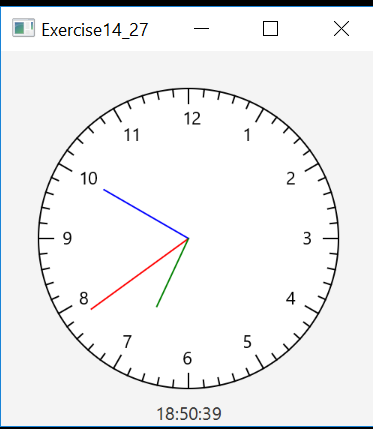### Create an Account

Home / Questions / Modify the ClockPane class in Section 14.12 of the course text to draw the clock with more...

# Modify the ClockPane class in Section 14.12 of the course text to draw the clock with more...

Detailed Clock (same as Programming Exercise 14.27, page 591)

Modify the ClockPane class in Section 14.12 of the course text to draw the clock with more details on hours and minutes, as shown in Figure 14.52a, on page 591.
The Clock Pane class that we have to modify is given below:

import java.util.Calendar;
import java.util.GregorianCalendar;
import javafx.scene.layout.Pane;
import javafx.scene.paint.Color;
import javafx.scene.shape.Circle;
import javafx.scene.shape.Line;
import javafx.scene.text.Text;

public class ClockPane extends Pane {
private int hour;
private int minute;
private int second;
/** Construct a default clock with the current time*/
public ClockPane() {
setCurrentTime();
}

/** Construct a clock with specified hour, minute, and second */
public ClockPane(int hour, int minute, int second) {
this.hour = hour;
this.minute = minute;
this.second = second;
}

/** Return hour */
public int getHour() {
return hour;
}

/** Set a new hour */
public void setHour(int hour) {
this.hour = hour;
paintClock();
}

/** Return minute */
public int getMinute() {
return minute;
}

/** Set a new minute */
public void setMinute(int minute) {
this.minute = minute;
paintClock();
}

/** Return second */
public int getSecond() {
return second;
}

/** Set a new second */
public void setSecond(int second) {
this.second = second;
paintClock();
}
/* Set the current time for the clock */
public void setCurrentTime() {
// Construct a calendar for the current date and time
Calendar calendar = new GregorianCalendar();

// Set current hour, minute and second
this.hour = calendar.get(Calendar.HOUR_OF_DAY);
this.minute = calendar.get(Calendar.MINUTE);
this.second = calendar.get(Calendar.SECOND);
paintClock(); // Repaint the clock
}
/** Paint the clock */
private void paintClock() {
// Initialize clock parameters
Math.min(getWidth(), getHeight()) * 0.8 * 0.5;
double centerX = getWidth() / 2;
double centerY = getHeight() / 2;

// Draw circle
Circle circle = new Circle(centerX, centerY, clockRadius);
circle.setFill(Color.WHITE);
circle.setStroke(Color.BLACK);
Text t1 = new Text(centerX - 5, centerY - clockRadius + 12, "12");
Text t2 = new Text(centerX - clockRadius + 3, centerY + 5, "9");
Text t3 = new Text(centerX + clockRadius - 10, centerY + 3, "3");
Text t4 = new Text(centerX - 3, centerY + clockRadius - 3, "6");
// Draw second hand
double sLength = clockRadius * 0.8;
double secondX = centerX + sLength *
Math.sin(second * (2 * Math.PI / 60));
double secondY = centerY - sLength *
Math.cos(second * (2 * Math.PI / 60));
Line sLine = new Line(centerX, centerY, secondX, secondY);
sLine.setStroke(Color.RED);

// Draw minute hand
double mLength = clockRadius * 0.65;
double xMinute = centerX + mLength *
Math.sin(minute * (2 * Math.PI / 60));
double minuteY = centerY - mLength *
Math.cos(minute * (2 * Math.PI / 60));
Line mLine = new Line(centerX, centerY, xMinute, minuteY);
mLine.setStroke(Color.BLUE);
// Draw hour hand
double hLength = clockRadius * 0.5;
double hourX = centerX + hLength *
Math.sin((hour % 12 + minute / 60.0) * (2 * Math.PI / 12));
double hourY = centerY - hLength *
Math.cos((hour % 12 + minute / 60.0) * (2 * Math.PI / 12));
Line hLine = new Line(centerX, centerY, hourX, hourY);
hLine.setStroke(Color.GREEN);
getChildren().clear();
getChildren().addAll(circle, t1, t2, t3, t4, sLine, mLine, hLine);
}
@Override
public void setWidth(double width) {
super.setWidth(width);
paintClock();
}
@Override
public void setHeight(double height) {
super.setHeight(height);
paintClock();
}
}

Modify the above code to add more details on hour and minutes like this:we have to add the numbers and lines in tha clock.Subscribe To Get Solution